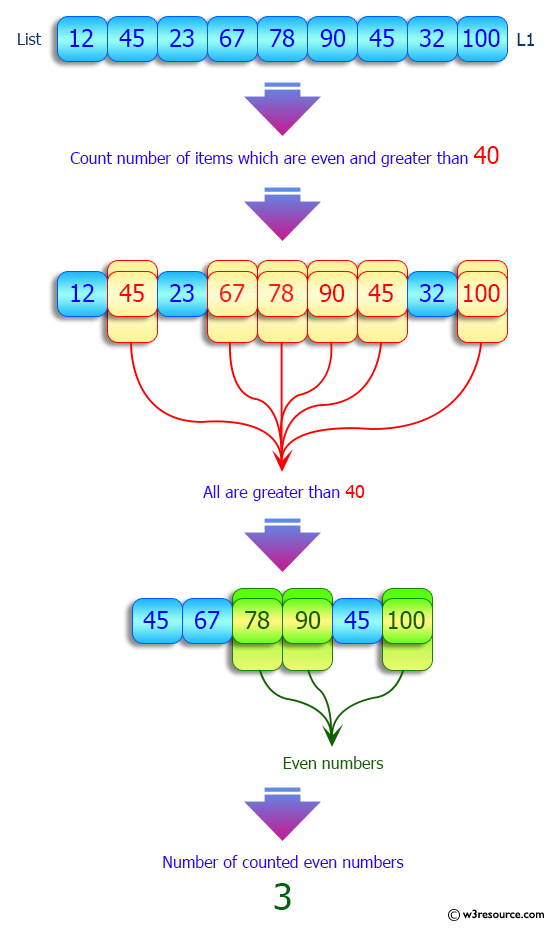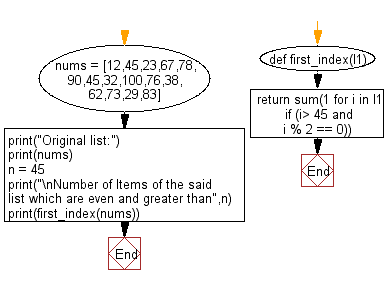﻿ Python: Get the items from a given list with specific condition - w3resource# Python: Get the items from a given list with specific condition

## Python List: Exercise - 164 with Solution

Write a Python program to get the items from a given list with specific condition.

Number of Items of the following list which are even and greater than 73
[12,45,23,67,78,90,45,32,100,76,38,62,73,29,83]
Output:
5

Sample Solution:

Python Code:

``````def first_index(l1):
return sum(1 for i in l1 if (i> 45 and i % 2 == 0))

nums = [12,45,23,67,78,90,45,32,100,76,38,62,73,29,83]
print("Original list:")
print(nums)
n = 45
print("\nNumber of Items of the said list which are even and greater than",n)
print(first_index(nums))
```
```

Sample Output:

```Original list:
[12, 45, 23, 67, 78, 90, 45, 32, 100, 76, 38, 62, 73, 29, 83]

Number of Items of the said list which are even and greater than 45
5
```

Pictorial Presentation:Flowchart:## Visualize Python code execution:

The following tool visualize what the computer is doing step-by-step as it executes the said program:

Python Code Editor:

Have another way to solve this solution? Contribute your code (and comments) through Disqus.

What is the difficulty level of this exercise?

Test your Python skills with w3resource's quiz

﻿

## Python: Tips of the Day

Floor Division:

When we speak of division we normally mean (/) float division operator, this will give a precise result in float format with decimals.

For a rounded integer result there is (//) floor division operator in Python. Floor division will only give integer results that are round numbers.

```print(1000 // 300)
print(1000 / 300)```

Output:

```3
3.3333333333333335```